# Phase-field model for crystallization in alkali disilicate glasses; Li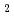O-2SiO, NaO-2SiOand KO-2SiOThis study developed phase-field method (PFM) technique in oxide melt system by using a new mobility coefficient (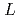). The crystal growth rates (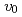) obtained by the PFM calculation with the constantwere comparable to the thermodynamic driving force in normal growth model. The temperature dependence of thewas determined from the experimental crystal growth rates and the. Using the determined, the crystal growth rates (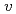) in alkali disilicate glasses, LiO-2SiO, NaO-2SiOand KO-2SiOwere simulated. The temperature dependence of thewas qualitatively and quantitatively so similar that the PFM calculation results demonstrated the validity of the. Especially, theobtained by the PFM calculation appeared the rapid increase just below the thermodynamic melting point (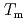) and the steep peak at around-100 K. Additionally, as the temperature decreased, theapparently approached zero ms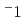, which is limited by therepresenting the interface jump process. Furthermore, we implemented the PFM calculation for the variation of the parameter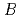in the. As theincreased from zero to two, the peak of thebecame steeper and the peak temperature of theshifted to the high temperature side. The parameters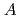andin theincreased exponentially and decreased linearly as the atomic number of the alkali metal increased due to the ionic potential, respectively. This calculation revealed that theandin thewere close and reasonable for each other.